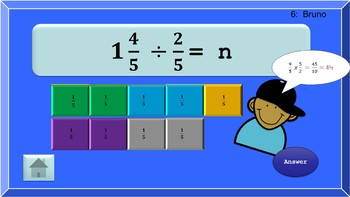# Fraction Operations Celebrity SquaresSubject
Resource Type
File Type

Zip

(12 MB|18 pages)
Standards
• Product Description
• Standards
This is a throwback of the old "Hollywood Squares" game show. Now the celebrities ask questions about adding, subtracting, multiplying, and dividing fractions using interactive models. Just divide the class into two teams, the X's and the O's. They'll love the original, fast-paced music and the exciting animations. They'll also love adding in the X's and O's live during the fast paced game. You'll love the five minutes of prep for this PowerPoint based platform and the alignment to the Common Core State Standards for fifth grade. Make sure your PowerPoint software is 2013 or newer and macro enabled. Have fun.
Solve real world problems involving division of unit fractions by non-zero whole numbers and division of whole numbers by unit fractions, e.g., by using visual fraction models and equations to represent the problem. For example, how much chocolate will each person get if 3 people share 1/2 lb of chocolate equally? How many 1/3-cup servings are in 2 cups of raisins?
Interpret division of a whole number by a unit fraction, and compute such quotients. For example, create a story context for 4 ÷ (1/5), and use a visual fraction model to show the quotient. Use the relationship between multiplication and division to explain that 4 ÷ (1/5) = 20 because 20 × (1/5) = 4.
Interpret division of a unit fraction by a non-zero whole number, and compute such quotients. For example, create a story context for (1/3) ÷ 4, and use a visual fraction model to show the quotient. Use the relationship between multiplication and division to explain that (1/3) ÷ 4 = 1/12 because (1/12) × 4 = 1/3.
Solve real world problems involving multiplication of fractions and mixed numbers, e.g., by using visual fraction models or equations to represent the problem.
Explaining why multiplying a given number by a fraction greater than 1 results in a product greater than the given number (recognizing multiplication by whole numbers greater than 1 as a familiar case); explaining why multiplying a given number by a fraction less than 1 results in a product smaller than the given number; and relating the principle of fraction equivalence 𝘢/𝘣 = (𝘯×𝘢)/(𝘯×𝘣) to the effect of multiplying 𝘢/𝘣 by 1.
Total Pages
18 pages
Included
Teaching Duration
40 minutes
Report this Resource to TpT
Reported resources will be reviewed by our team. Report this resource to let us know if this resource violates TpT’s content guidelines.